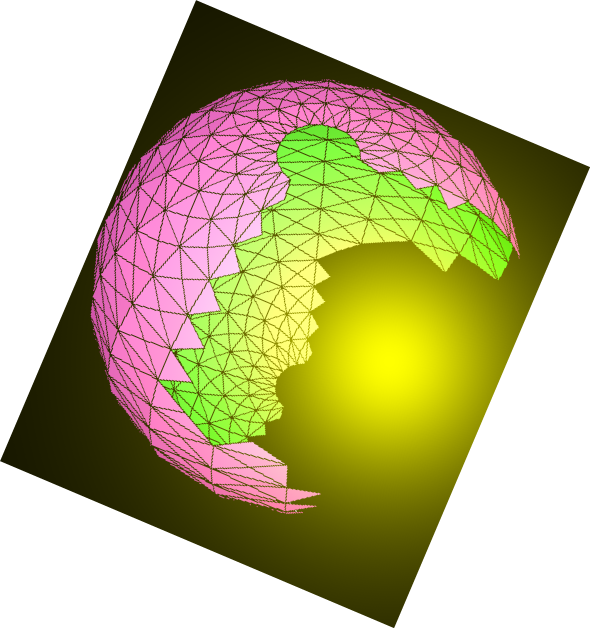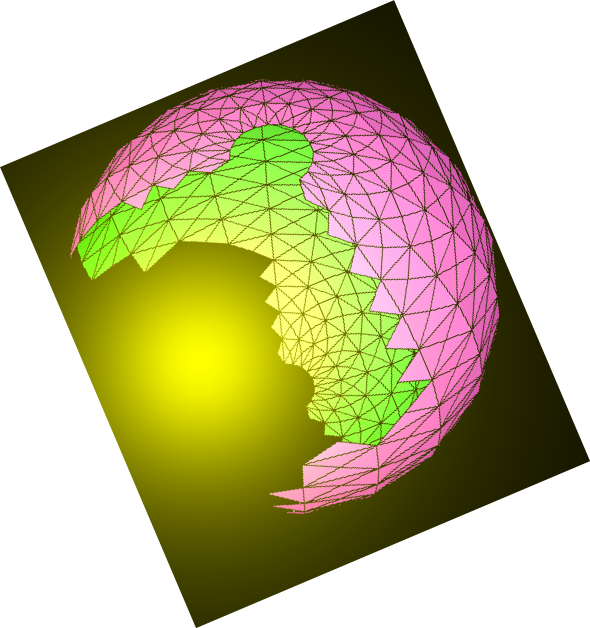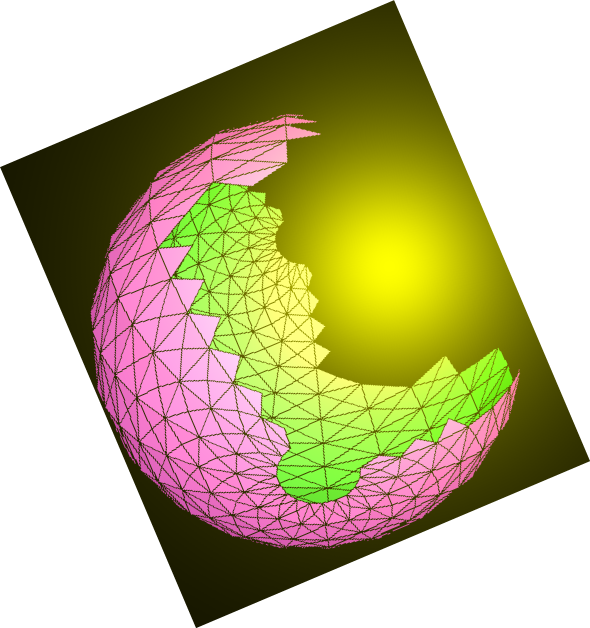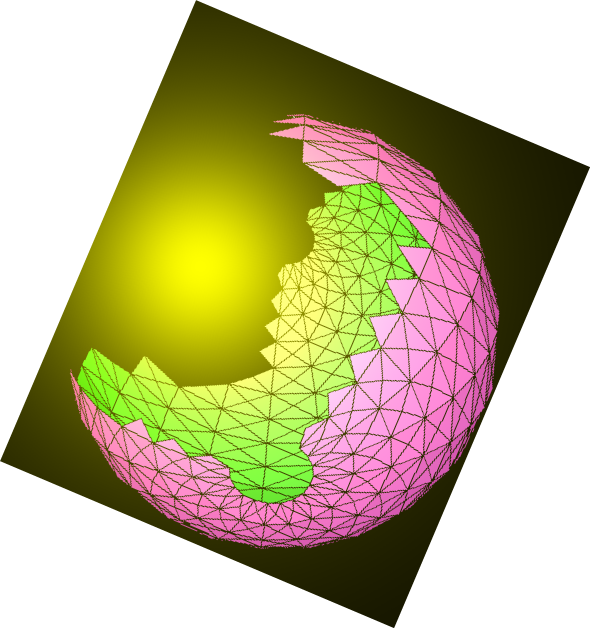﻿ JavaScript & MathScript Interactive Document Generator-Demo-01# N.S.B. Cosmic Center# JavaScript & MathScript Interactive Document Generator-Demo-01

This document is generated by N.S.B. JavaScript & MathScript Interactive Document Generator version 001.

Introduction to N.S.B. JavaScript & MathScript Interactive Document Generator

Using JavaScript

In order to have this program interpret your JavaScript code, you can write the code at the input line, with correct JavaScript syntax, then hit the button . For instance, the following code sequence starts by introducing a couple of variables, then execute various JavaScript operations on them. Remember to hit the proper button--we shall return to the other buttons later.

###### output(3)=> -2.315

Notice our use, in the last operation, of the function \$() which denotes the last JavaScript result. In general, we use \$(n) with positive integer n to denote the JavaScript output(n); we use \$(-1) as well as \$() to denote the last result, other negative arguments to count backwards. For instance, consider these:

###### output(5)=> 0

We shall return in other demos to various aspects of JavaScript. For now, let us introduce MathScript, and the associated button.

Using MathScript

In this interactive program, we have used JavaScript to implement an interpreter of code very much like the wellknown Mathematica program. However, our implementation is not exactly similar to the Mathematica language--in fact, various aspects mark some improvement.

If you want your input code to be interpreted as MathScript, you must hit the button , and be sure that you're using the correct MathScript syntax. In general, whether you're using JavaScript or MathScript, this program would either alert you if you have sysntax errors, or it would not produce an output.

Notice that, like Mathematica, our MathScript functions utilize square brackets, while we use curly bracket to represent arrays or lists, and ordinary brackets for groupings. Consider the following sequence of code being executed as MathScript:

###### output(8)=> -4.658

In the above sequence, we started by introducing a list {a,b,c}. The first output returns the same list unmodified. Then we refer to that output by using the symbol %. (In MathScript, we use % to denote the last output, %% to the one before last, %%% to the one twice before, and so on; we also use %1, %2, etc. to denote output(1), output(2), etc. ) We have used /. to denote the replacement operation. This is followed by a list of replacement rules (using the operator =>). Finally, we applied the operation of multiplication ('mult') on the elements of the resulting list.

The above account should have given you some taste for the usage of this program that implements JavaScript as well as MathScript. Forthcoming documents will go into many more demonstrations. However, you must know that the whole document you're reading is prepared by the same program. The HTML elements are all executed at the input line. This is done by writing the proper HTML code then hitting the button . You can learn more of this later on.

###### NextHome
N.S.B. Cosmic Center